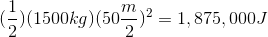# AP Physics B : Understanding Kinetic Energy

## Example Questions

### Example Question #1 : Work, Energy, And Power

A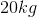shopping cart is traveling at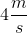in a bumpy parking lot. The constant friction force on the cart is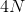. How far does the cart travel before coming a complete stop?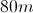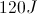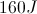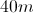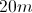Explanation:

We can use work and energy to solve this problem. First, solve for the initial kinetic energy of the shopping cart, using its initial velocity: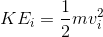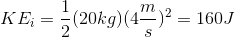The final kinetic energy will be zero, since the cart will come to a complete stop. Work is equal to the change in mechanical energy (in this case kinetic energy).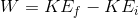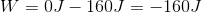Work can also be written as the product of a force acting over a distance. In this case, the constant force is friction.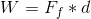Use the calculated value of work and the force of friction to solve for the distance. Remember that force of friction will be negative since it acts in the opposite direction of the motion.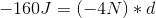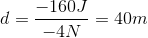### Example Question #1 : Work, Energy, And Power

If an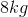ball is traveling with a horizontal velocity of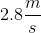and a vertical velocity of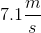, what is its kinetic energy?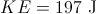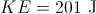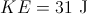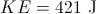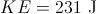Explanation:

We know that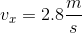and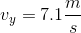. First, calculate the translational velocity by using the Pythagorean Theorem.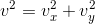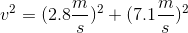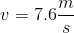We can use this to find the total kinetic energy.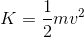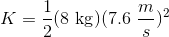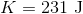### Example Question #1 : Understanding Kinetic Energy

A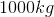car is initially driving at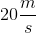on a flat street. The car then speeds up to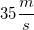. What is the total change in kinetic energy of the car?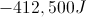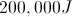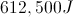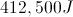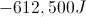Explanation:

First, we calculate the initial kinetic energy of the car: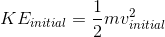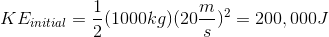Then, calculate the final kinetic energy after the car has reached a velocity of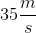: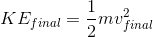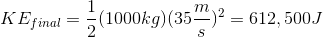To find the change in kinetic energy, we subtract the initial energy from the final energy.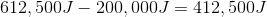This answer in positive because the car gains energy when it increases its velocity.

### Example Question #1 : Understanding Kinetic Energy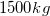car starting from rest accelerates at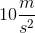for five seconds. What is the kinetic energy of this car after this period of acceleration?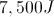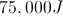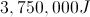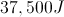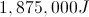Explanation:

The first thing we need to find is the final velocity of the car. We know it starts from rest and has an acceleration offor five seconds. We can calculate the final velocity using these values and the appropriate kinematics equation.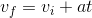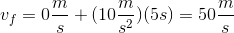The formula for kinetic energy is: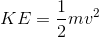We can use the calculated final velocity and the mass of the car to determine its final kinetic energy.